# Category: representations

If you take two Fischer involutions in the monster (elements of conjugacy class 2A) and multiply them, the resulting element surprisingly belongs to one of just 9 conjugacy classes:

1A,2A,2B,3A,3C,4A,4B,5A or 6A

The orders of these elements are exactly the dimensions of the fundamental root for the extended $E_8$ Dynkin diagram.

This is the content of John McKay’s E(8)-observation : there should be a precise relation between the nodes of the extended Dynkin diagram and these 9 conjugacy classes in such a way that the order of the class corresponds to the component of the fundamental root. More precisely, one conjectures the following correspondence: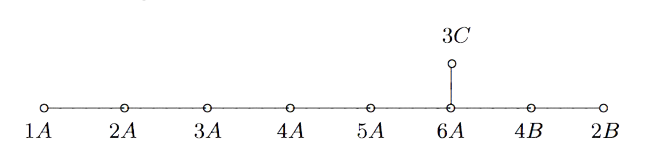John Duncan found such a connection by considering carefully the corresponding moonshine groups and their inter-relation. For more on this, look at the old post E8 from moonshine groups. The extended Dynkin diagram with these moonshine groups as vertices is: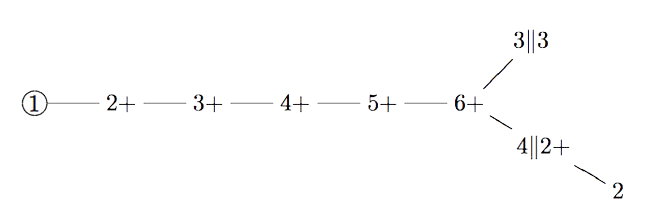Duncan does this by assigning numbers to moonshine groups: the dimension is the order of the corresponding monster element and the valency is one more than the copies of $C_2$ generated by the Atkin-Lehner involutions in the moonshine group.

One might ask whether there is a graph on all 171 moonshine groups, compatible with the valencies of every vertex.

Now, even for the 9 groups in McKay’s question, the valencies do not determine the graph uniquely and Duncan proceeds with an ad hoc condition on the edges.

There is a partition on the 9 groups by the property whether or not the index of the intersection with $\Gamma_0(2)$ is at most two. Then Duncan declares that there cannot be an edge between two groups belonging to the same class.

His motivation for this property comes from classical McKay-correspondence for the binary icosahedral group (where the vertices correspond to simple representations $S$, and the edges from $S$ to factors of $S \otimes V_2$, where $V_2$ is the restriction of the standard $2$-dimensional simple for $SU(2)$).

Of the $9$ simples there are only $4$ faithful ones, $5$ come from simples of $A_5$. Because $\Gamma_0(2)$ is a subgroup of the modular group of index 2, he then views $\Gamma_0(2)$ as similar to the subgroup $A_5$ in the binary icosahedral group, and declares a moonshine group to be faithful if its index in the intersection with $\Gamma_0(2)$ is at most two.

One might ask whether there is another, more natural, definition for having an edge (or multiple ones) between arbitrary moonshine groups.

And, what is the full graph on the 171 groups?

Today, Samuel Dehority, Xavier Gonzalez, Neekon Vafa and Roger Van Peski arXived their paper Moonshine for all finite groups.

Originally, Moonshine was thought to be connected to the Monster group. McKay and Thompson observed that the first coefficients of the normalized elliptic modular invariant

$J(\tau) = q^{-1} + 196884 q + 21493760 q^2 + 864229970 q^3 + \ldots$

could be written as sums of dimensions of the first few irreducible representations of the monster group:

$1=1,~\quad 196884=196883+1,~\quad 21493760=1+196883+21296876,~\quad …$

Soon it transpired that there ought to be an infinite dimensional graded vectorspace, the moonshine module

$V^{\sharp} = \bigoplus_{n=-1}^{\infty}~V^{\sharp}_n$

with every component $V^{\sharp}_n$ being a representation of the monster group $\mathbb{M}$ of which the dimension coincides with the coefficient of $q^n$ in $J(\tau)$.

It only got better, for any conjugacy class $[ g ]$ of the monster, if you took the character series

$T_g(\tau) = \sum_{n=-1}^{\infty} Tr(g | V^{\sharp}_n) q^n$

you get a function invariant under the action of the subgroup

$\Gamma_0(n) = \{ \begin{bmatrix} a & b \\ c & d \end{bmatrix}~:~c = 0~mod~n \}$

acting via transformations $\tau \mapsto \frac{a \tau + b}{c \tau + d}$ on the upper half plane where $n$ is the order of $g$ (or, for the experts, almost).

Soon, further instances of ‘moonshine’ were discovered for other simple groups, the unifying feature being that one associates to a group $G$ a graded representation $V$ such that the character series of this representation for an element $g \in G$ is an invariant modular function with respect to the subgroup $\Gamma_0(n)$ of the modular group, with $n$ being the order of $g$.

Today, this group of people proved that there is ‘moonshine’ for any finite group whatsoever.

They changed the definition of moonshine slightly to introduce the notion of moonshine of depth $d$ which meant that they want the dimension sequence of their graded module to be equal to $J(\tau)$ under the action of the normalized $d$-th Hecke operator, which means equal to

$\sum_{ac=d,0 \leq b < c} J(\frac{a \tau + b}{c})$
as they are interested in the asymptotic behaviour of the components $V_n$ with respect to the regular representation of $G$.

What baffled me was their much weaker observation (remark 2) saying that you get ‘moonshine’ in the form described above, that is, a graded representation $V$ such that for every $g \in G$ you get a character series which is invariant under $\Gamma_0(n)$ with $n=ord(g)$ (and no smaller divisor of $n$), for every finite group $G$.

And, more importantly, you can explain this to any student taking a first course in group theory as all you need is Cayley’s theorem stating that any finite group is a subgroup of some symmetric group $S_n$.

Here’s the idea: take the original monster-moonshine module $V^{\sharp}$ but forget all about the action of $\mathbb{M}$ (that is, consider it as a plain vectorspace) and consider the graded representation

$V = (V^{\sharp})^{\otimes n}$

with the natural action of $S_n$ on the tensor product.

Now, embed a la Cayley $G$ into $S_n$ then you know that the order of $g \in G$ is the least common multiple of the cycle lengths of the permutation it it send to. Now, it is fairly trivial to see that the character series of $V$ with respect to $g$ (having cycle lengths $(k_1,k_2,\dots,k_l)$, including cycles of length one) is equal to the product

$J(k_1 \tau) J(k_2 \tau) \dots J(k_l \tau)$

which is invariant under $\Gamma_0(n)$ with $n = lcm(k_i)$ (but no $\Gamma_0(m)$ with $m$ a proper divisor of $n$).

For example, for $G=S_4$ we have as character series of $(V^{\sharp})^{\otimes 4}$

$(1)(2)(3)(4) \mapsto J(\tau)^4$

$(12)(3)(4) \mapsto J(2 \tau) J(\tau)^2$

$(12)(34) \mapsto J(2 \tau)^2$

$(123)(4) \mapsto J(3 \tau) J(\tau)$

$(1234) \mapsto J(4 \tau)$

Clearly, the main results of the paper are much more subtle, but I’m already happy with this version of ‘moonshine for everyone’!

Please allow for a couple of end-of-semester bluesy ramblings. I just finished grading the final test of the last of five courses I lectured this semester.

Most of them went, I believe, rather well.

As always, it was fun to teach an introductory group theory course to second year physics students.

Personally, I did enjoy our Lie theory course the most, given for a mixed public of both mathematics and physics students. We did the spin-group $SU(2)$ and its connection with $SO_3(\mathbb{R})$ in gruesome detail, introduced the other classical groups, and proved complete reducibility of representations. The funnier part was applying this to the $U(1) \times SU(2) \times SU(3)$-representation of the standard model and its extension to the $SU(5)$ GUT.

Ok, but with a sad undertone, was the second year course on representations of finite groups. Sad, because it was the last time I’m allowed to teach it. My younger colleagues decided there’s no place for RT on the new curriculum.

Soit.

The final lecture is often an eye-opener, or at least, I hope it is/was.

Here’s the idea: someone whispers in your ear that there might be a simple group of order $60$. Armed with only the Sylow-theorems and what we did in this course we will determine all its conjugacy classes, its full character table, and finish proving that this mysterious group is none other than $A_5$.

Right now I’m just a tad disappointed only a handful of students came close to solving the same problem for order $168$ this afternoon.

Clearly, I gave them ample extra information: the group only has elements of order $1,2,3,4$ and $7$ and the centralizer of one order $2$ element is the dihedral group of order $8$. They had to determine the number of distinct irreducible representations, that is, the number of conjugacy classes. Try it yourself (Solution at the end of this post).

For months I felt completely deflated on Tuesday nights, for I had to teach the remaining two courses on that day.

There’s this first year Linear Algebra course. After teaching for over 30 years it was a first timer for me, and probably for the better. I guess 15 years ago I would have been arrogant enough to insist that the only way to teach linear algebra properly was to do representations of quivers…

Now, I realise that linear algebra is perhaps the only algebra course the majority of math-students will need in their further career, so it is best to tune its contents to the desires of the other colleagues: inproducts, determinants as volumes, Markov-processes and the like.

There are thousands of linear algebra textbooks, the one feature they all seem to lack is conciseness. What kept me going throughout this course was trying to come up with the shortest proofs ever for standard results. No doubt, next year the course will grow on me.

Then, there was a master course on algebraic geometry (which was supposed to be on scheme theory, moduli problems such as the classification of fat points (as in the car crash post, etale topology and the like) which had a bumpy start because class was less prepared on varieties and morphisms than I had hoped for.

Still, judging on the quality of the papers students are beginning to hand in (today I received one doing serious stuff with stacks) we managed to cover a lot of material in the end.

I’m determined to teach that first course on algebraic geometry myself next year.

Which brought me wondering about the ideal content of such a course.

Half a decade ago I wrote a couple of posts such as Mumford’s treasure map, Grothendieck’s functor of points, Manin’s geometric axis and the like, which are still quite readable.

In the functor of points-post I referred to a comment thread Algebraic geometry without prime ideals at the Secret Blogging Seminar.

As I had to oversee a test this afternoon, I printed out all comments (a full 29 pages!) and had a good time reading them. At the time I favoured the POV advocated by David Ben-Zvi and Jim Borger (functor of points instead of locally ringed schemes).

Clearly they are right, but then so was I when I thought the ‘right’ way to teach linear algebra was via quiver-representations…

We’ll see what I’ll try out next year.

You may have wondered about the title of this post. It’s derived from a paper Raf Bocklandt (of the Korteweg-de Vries Institute in Amsterdam) arXived some days ago: Reflections in a cup of coffee, which is an extended version of a Brouwer-lecture he gave. Raf has this to say about the Brouwer fixed-point theorem.

“The theorem is usually explained in worldly terms by looking at a cup of coffee. In this setting it states that no matter how you stir your cup, there will always be a point in the liquid that did not change position and if you try to move that part by further stirring you will inevitably move some other part back into its original position. Legend even has it that Brouwer came up with the idea while stirring in a real cup, but whether this is true we’ll never know. What is true however is that Brouwers refections on the topic had a profound impact on mathematics and would lead to lots of new developments in geometry.”

I wish you all a pleasant end of 2016 and a much better 2017.

As to the 168-solution: Sylow says there are 8 7-Sylows giving 48 elements of order 7. The centralizer of each of them must be $C_7$ (given the restriction on the order of elements) so two conjugacy classes of them. Similarly each conjugacy class of an order 3 element must contain 56 elements. There is one conjugacy class of an order 2 element having 21 elements (because the centralizer is $D_4$) giving also a conjugacy class of an order 4 element consisting of 42 elements. Together with the identity these add up to 168 so there are 6 irreducible representations.

Santa gave me 3000 Euros to spend on books. One downside: I have to give him my wish-list before monday. So, I’d better get started. Clearly, any further suggestions you might have will be much appreciated, either in the comments below or more directly via email.

Today I’ll focus on my own interests: algebraic geometry, non-commutative geometry and representation theory. I do own a fair amount of books already which accounts for the obvious omissions in the lists below (such as Hartshorne, Mumford or Eisenbud-Harris in AG, Fulton-Harris in RT or the ‘bibles’ in NCG).

[section_title text=”Algebraic geometry”]Here, I base myself on (and use quotes from) the excellent answer by Javier Alvarez to the MathOverflow post Best Algebraic Geometry text book? (other than Hartshorne).

In no particular order:

Lectures on Curves, Surfaces and Projective Varieties by Ettore Carletti, Dionisio Gallarati, and Giacomo Monti Bragadin and Mauro C. Beltrametti.
“which starts from the very beginning with a classical geometric style. Very complete (proves Riemann-Roch for curves in an easy language) and concrete in classic constructions needed to understand the reasons about why things are done the way they are in advanced purely algebraic books. There are very few books like this and they should be a must to start learning the subject. (Check out Dolgachev’s review.)”

A Royal Road to Algebraic Geometry by Audun Holme. “This new title is wonderful: it starts by introducing algebraic affine and projective curves and varieties and builds the theory up in the first half of the book as the perfect introduction to Hartshorne’s chapter I. The second half then jumps into a categorical introduction to schemes, bits of cohomology and even glimpses of intersection theory.”

Liu Qing – “Algebraic Geometry and Arithmetic Curves”. “It is a very complete book even introducing some needed commutative algebra and preparing the reader to learn arithmetic geometry like Mordell’s conjecture, Faltings’ or even Fermat-Wiles Theorem.”

Görtz; Wedhorn – Algebraic Geometry I, Schemes with Examples and Exercises. labeled ‘the best on schemes’ by Alvarez. “Tons of stuff on schemes; more complete than Mumford’s Red Book. It does a great job complementing Hartshorne’s treatment of schemes, above all because of the more solvable exercises.”

Kollár – Lectures on Resolution of Singularities. “Great exposition, useful contents and examples on topics one has to deal with sooner or later.”

Kollár; Mori – Birational Geometry of Algebraic Varieties. “Considered as harder to learn from by some students, it has become the standard reference on birational geometry.”

And further, as a follow-up on their previous book on the computational side of AG:

Using Algebraic Geometry by Cox, Little and O’Shea.

[section_title text=”Non-commutative geometry”]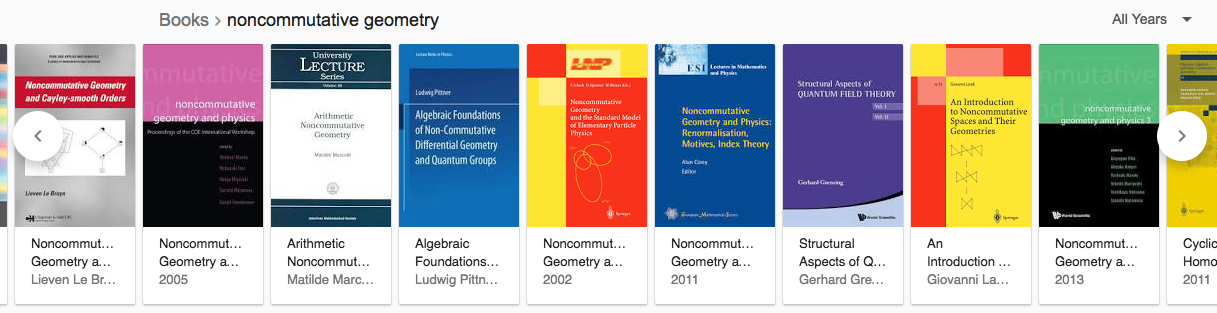Noncommutative Geometry and Particle Physics by Walter van Suijlekom. Blurb: “This book provides an introduction to noncommutative geometry and presents a number of its recent applications to particle physics. It is intended for graduate students in mathematics/theoretical physics who are new to the field of noncommutative geometry, as well as for researchers in mathematics/theoretical physics with an interest in the physical applications of noncommutative geometry. In the first part, we introduce the main concepts and techniques by studying finite noncommutative spaces, providing a “light” approach to noncommutative geometry. We then proceed with the general framework by defining and analyzing noncommutative spin manifolds and deriving some main results on them, such as the local index formula. In the second part, we show how noncommutative spin manifolds naturally give rise to gauge theories, applying this principle to specific examples. We subsequently geometrically derive abelian and non-abelian Yang-Mills gauge theories, and eventually the full Standard Model of particle physics, and conclude by explaining how noncommutative geometry might indicate how to proceed beyond the Standard Model.”

An Invitation To Noncommutative Geometry by Matilde Marcolli. Blurb: “This is the first existing volume that collects lectures on this important and fast developing subject in mathematics. The lectures are given by leading experts in the field and the range of topics is kept as broad as possible by including both the algebraic and the differential aspects of noncommutative geometry as well as recent applications to theoretical physics and number theory.”

Noncommutative Geometry and Physics: Renormalisation, Motives, Index Theory. Blurb: “This collection of expository articles grew out of the workshop “Number Theory and Physics” held in March 2009 at The Erwin Schrödinger International Institute for Mathematical Physics, Vienna. The common theme of the articles is the influence of ideas from noncommutative geometry (NCG) on subjects ranging from number theory to Lie algebras, index theory, and mathematical physics. Matilde Marcolli’s article gives a survey of relevant aspects of NCG in number theory, building on an introduction to motives for beginners by Jorge Plazas and Sujatha Ramdorai.”

Feynman Motives by Matilde Marcolli. Blurb: “This book presents recent and ongoing research work aimed at understanding the mysterious relation between the computations of Feynman integrals in perturbative quantum field theory and the theory of motives of algebraic varieties and their periods. One of the main questions in the field is understanding when the residues of Feynman integrals in perturbative quantum field theory evaluate to periods of mixed Tate motives.” But then, check out Matilde’s recent FaceBook status-update.

[section_title text=”Representation theory”]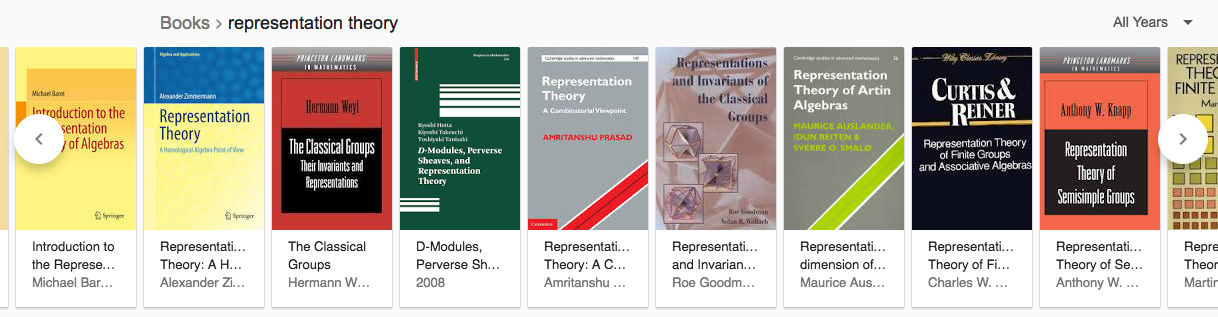An Introduction to the Langlands Program by J. Bernstein (editor). Blurb: “This book presents a broad, user-friendly introduction to the Langlands program, that is, the theory of automorphic forms and its connection with the theory of L-functions and other fields of mathematics. Each of the twelve chapters focuses on a particular topic devoted to special cases of the program. The book is suitable for graduate students and researchers.”

Representation Theory of Finite Groups: An Introductory Approach by Benjamin Steinberg.

Representation Theory of Finite Monoids by Benjamin Steinberg. Blurb: “This first text on the subject provides a comprehensive introduction to the representation theory of finite monoids. Carefully worked examples and exercises provide the bells and whistles for graduate accessibility, bringing a broad range of advanced readers to the forefront of research in the area. Highlights of the text include applications to probability theory, symbolic dynamics, and automata theory. Comfort with module theory, a familiarity with ordinary group representation theory, and the basics of Wedderburn theory, are prerequisites for advanced graduate level study.”

How am I doing? 914 dollars…

Way to go, same exercise tomorrow. Again, suggestions/warnings welcome!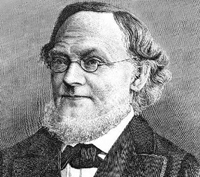A standard Grassmannian $Gr(m,V)$ is the manifold having as its points all possible $m$-dimensional subspaces of a given vectorspace $V$. As an example, $Gr(1,V)$ is the set of lines through the origin in $V$ and therefore is the projective space $\mathbb{P}(V)$. Grassmannians are among the nicest projective varieties, they are smooth and allow a cell decomposition.

A quiver $Q$ is just an oriented graph. Here’s an example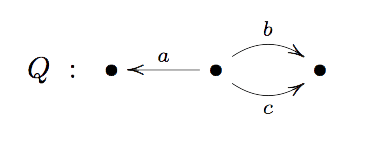A representation $V$ of a quiver assigns a vector-space to each vertex and a linear map between these vertex-spaces to every arrow. As an example, a representation $V$ of the quiver $Q$ consists of a triple of vector-spaces $(V_1,V_2,V_3)$ together with linear maps $f_a~:~V_2 \rightarrow V_1$ and $f_b,f_c~:~V_2 \rightarrow V_3$.

A sub-representation $W \subset V$ consists of subspaces of the vertex-spaces of $V$ and linear maps between them compatible with the maps of $V$. The dimension-vector of $W$ is the vector with components the dimensions of the vertex-spaces of $W$.

This means in the example that we require $f_a(W_2) \subset W_1$ and $f_b(W_2)$ and $f_c(W_2)$ to be subspaces of $W_3$. If the dimension of $W_i$ is $m_i$ then $m=(m_1,m_2,m_3)$ is the dimension vector of $W$.

The quiver-analogon of the Grassmannian $Gr(m,V)$ is the Quiver Grassmannian $QGr(m,V)$ where $V$ is a quiver-representation and $QGr(m,V)$ is the collection of all possible sub-representations $W \subset V$ with fixed dimension-vector $m$. One might expect these quiver Grassmannians to be rather nice projective varieties.

However, last week Markus Reineke posted a 2-page note on the arXiv proving that every projective variety is a quiver Grassmannian.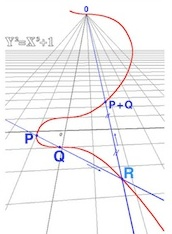Let’s illustrate the argument by finding a quiver Grassmannian $QGr(m,V)$ isomorphic to the elliptic curve in $\mathbb{P}^2$ with homogeneous equation $Y^2Z=X^3+Z^3$.

Consider the Veronese embedding $\mathbb{P}^2 \rightarrow \mathbb{P}^9$ obtained by sending a point $(x:y:z)$ to the point

$(x^3:x^2y:x^2z:xy^2:xyz:xz^2:y^3:y^2z:yz^2:z^3)$

The upshot being that the elliptic curve is now realized as the intersection of the image of $\mathbb{P}^2$ with the hyper-plane $\mathbb{V}(X_0-X_7+X_9)$ in the standard projective coordinates $(x_0:x_1:\cdots:x_9)$ for $\mathbb{P}^9$.

To describe the equations of the image of $\mathbb{P}^2$ in $\mathbb{P}^9$ consider the $6 \times 3$ matrix with the rows corresponding to $(x^2,xy,xz,y^2,yz,z^2)$ and the columns to $(x,y,z)$ and the entries being the multiplications, that is

$$\begin{bmatrix} x^3 & x^2y & x^2z \\ x^2y & xy^2 & xyz \\ x^2z & xyz & xz^2 \\ xy^2 & y^3 & y^2z \\ xyz & y^2z & yz^2 \\ xz^2 & yz^2 & z^3 \end{bmatrix} = \begin{bmatrix} x_0 & x_1 & x_2 \\ x_1 & x_3 & x_4 \\ x_2 & x_4 & x_5 \\ x_3 & x_6 & x_7 \\ x_4 & x_7 & x_8 \\ x_5 & x_8 & x_9 \end{bmatrix}$$

But then, a point $(x_0:x_1: \cdots : x_9)$ belongs to the image of $\mathbb{P}^2$ if (and only if) the matrix on the right-hand side has rank $1$ (that is, all its $2 \times 2$ minors vanish). Next, consider the quiver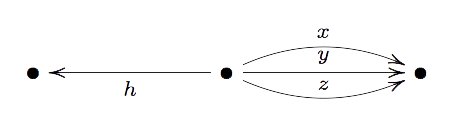and consider the representation $V=(V_1,V_2,V_3)$ with vertex-spaces $V_1=\mathbb{C}$, $V_2 = \mathbb{C}^{10}$ and $V_2 = \mathbb{C}^6$. The linear maps $x,y$ and $z$ correspond to the columns of the matrix above, that is

$$(x_0,x_1,x_2,x_3,x_4,x_5,x_6,x_7,x_8,x_9) \begin{cases} \rightarrow^x~(x_0,x_1,x_2,x_3,x_4,x_5) \\ \rightarrow^y~(x_1,x_3,x_4,x_6,x_7,x_8) \\ \rightarrow^z~(x_2,x_4,x_5,x_7,x_8,x_9) \end{cases}$$

The linear map $h~:~\mathbb{C}^{10} \rightarrow \mathbb{C}$ encodes the equation of the hyper-plane, that is $h=x_0-x_7+x_9$.

Now consider the quiver Grassmannian $QGr(m,V)$ for the dimension vector $m=(0,1,1)$. A base-vector $p=(x_0,\cdots,x_9)$ of $W_2 = \mathbb{C}p$ of a subrepresentation $W=(0,W_2,W_3) \subset V$ must be such that $h(x)=0$, that is, $p$ determines a point of the hyper-plane.

Likewise the vectors $x(p),y(p)$ and $z(p)$ must all lie in the one-dimensional space $W_3 = \mathbb{C}$, that is, the right-hand side matrix above must have rank one and hence $p$ is a point in the image of $\mathbb{P}^2$ under the Veronese.

That is, $Gr(m,V)$ is isomorphic to the intersection of this image with the hyper-plane and hence is isomorphic to the elliptic curve.

The general case is similar as one can view any projective subvariety $X \rightarrow \mathbb{P}^n$ as isomorphic to the intersection of the image of a specific $d$-uple Veronese embedding $\mathbb{P}^n \rightarrow \mathbb{P}^N$ with a number of hyper-planes in $\mathbb{P}^N$.